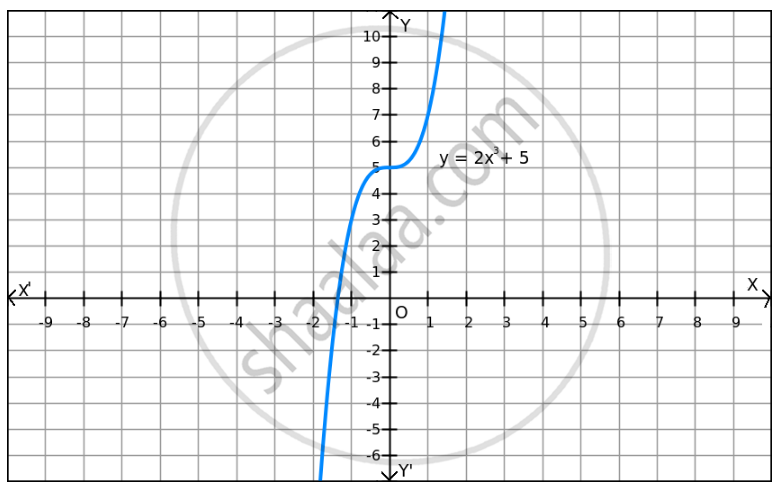PUC Karnataka Science Class 12Department of Pre-University Education, Karnataka
Share

F(X)=2x3 +5 on R . - PUC Karnataka Science Class 12 - Mathematics

Question

f(x)=2x3 +5 on R .

Solution

We can observe that f(x) increases when the values of x are increased and f(x) decreases when the values of x are decreased. Also, f(x) can be reduced by giving small values of x.

Similarly, f(x) can be enlarged by giving large values of x.

So, f(x) does not have a minimum or maximum value.Is there an error in this question or solution?

Video TutorialsVIEW ALL 

Solution F(X)=2x3 +5 on R . Concept: Graph of Maxima and Minima.
S